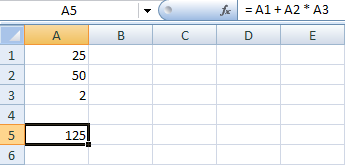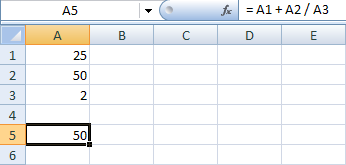# Combining Arithmetic Operators in Excel

The basic operators you've just met can be combined to make more complex calculations. For example, you can add to cells together, and multiply by a third one. Like this:

= A1 + A2 * A3

Or this:

= A1 + A2 - A3

And even this:

=SUM(A1:A9) * B1

In the above formula, we're asking Excel to add up the numbers in the cells A1 to A9, and then multiply the answer by B1. You'll get some practise with combining the operators shortly. But there's something you need to be aware of called Operator Precedence.

### Operator Precedence

Some of the operators you have just met are calculated before others. This is known as Operator Precedence. As an example, try this:

• Open a new Excel spreadsheet
• In cell A1 enter 25
• In cell A2 enter 50
• In cell A3 enter 2
Now click in cell A5 and enter the following formula:

=(A1 + A2) * A3

Hit the enter key on your keyboard, and you'll see an answer of 150.

The thing to pay attention to here is the brackets. When you place brackets around cell references, you section these cells off. Excel will then work out the answer to your formula inside of the brackets, A1 + A2 in our formula. Once it has the answer to whatever is inside of your round brackets, it will move on and calculate the rest of your formula. For us, this was multiply by 3. So Excel is doing this:

• Add up the A1 and A2 in between the round brackets
• Multiply that answer by A3

Now try this:

• Click inside A5 where your formula is
• Now click into the formula bar at the top
• Delete the two round brackets
• Hit the enter key on your keyboard
What answer did you get? The images below show the answers with brackets and without:

With BracketsWithout BracketsSo why did Excel give you two different answers? The reason it did so is because of operator precedence. Excel sees multiplication as more important than adding up, so it does that first. Without the brackets, our formula is this:

A1 + A2 * A3

You and I may work out the answer to that formula from left to right. So we'll add A1 + A2, and THEN multiply by A3. But because Excel sees multiplication as more important, it will do the calculation this way:

• Multiply A2 by A3 first

We have 50 in cell A2, and in cell A3 we have the number 2. When you multiply 50 by 2 you get 100. Add the 25 in cell A1 and the answer is 125.

When we used the brackets, we forced Excel to do the addition first:

(A1 + A2) * A3

Add the 25 in cell A1 to the 50 in cell A2 and your get 75. Now multiply by the 2 in cell A3 and you 150.

One answer is not more correct than the other. But because of operator precedence it meant that the multiplication got done first, then the addition. We had to used round brackets to tell Excel what we wanted doing first. Here's another example of operator precedence.

Substitute the asterisk symbol from your formula above with the division symbol. So instead of this:

= (A1 + A2) * A3

the formula will be this:

= (A1 + A2) / A3

When you hit the enter key on your keyboard, you should get an answer of 37.5.
Now click into cell A5, and then click into the formula bar. Delete the two round brackets, and hit the enter key again. What answer did you get this time? Here's the two images:

With the bracketsWithout the bracketsJust like multiplication, division is seen as more important than addition. So this will get done first. Without the brackets, Excel will first divide A2 by A3. When it has the answer, it will then add the A1. We used the round brackets to force Excel to calculate things differently. Hence the two different answers. One final example.

Change you formula in cell A5 to this:

= (A1 * A2) / A3

Hit the enter key, and you should get an answer of 625.

Again remove the brackets, and hit the enter key. You'll still have an answer of 625. That's because Excel treats multiplication the same as division: they have equal importance. When this happens, Excel will work out the answer from left to right.

Addition and subtraction are also seen as equal to each other. Try this formula in cell A5:

= A1 + A2 - A3

Now put some round brackets in. Try this first:

= (A1 + A2) - A3

And then see what happens when you try this:

= A1 + (A2 - A3)

Was there any difference? There shouldn't have been. You should have the same answer.

So keep Operator Precedence in mind - all sums are not treated equally!

To give you some practice with combination formulas, have a go at constructing the more complex Budget spreadsheet in the link below.

<--Back to the Excel Contents Page